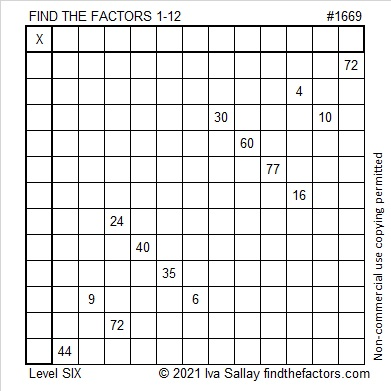# 1669 and Level 6

Contents

### Today’s Puzzle:

Some sets of clues in this puzzle have two possible common factors, and another set has three possible common factors. Don’t guess which ones to use, but use logic instead! Write the numbers 1 to 12 in both the first column and the top row so that those numbers and the given clues form a multiplication table.### Factors of 1669:

• 1669 is a prime number.
• Prime factorization: 1669 is prime.
• 1669 has no exponents greater than 1 in its prime factorization, so √1669 cannot be simplified.
• The exponent in the prime factorization is 1. Adding one to that exponent we get (1 + 1) = 2. Therefore 1669 has exactly 2 factors.
• The factors of 1669 are outlined with their factor pair partners in the graphic below.How do we know that 1669 is a prime number? If 1669 were not a prime number, then it would be divisible by at least one prime number less than or equal to √1669. Since 1669 cannot be divided evenly by 2, 3, 5, 7, 11, 13, 17, 19, 23, 29, 31, or 37, we know that 1669 is a prime number.

### More About the Number 1669:

1669 is the sum of two squares:
38² + 15² = 1669.

1669 is the hypotenuse of a primitive Pythagorean triple:
1140-1219-1669, calculated from 2(38)(15), 38² – 15², 38² + 15².

Here’s another way we know that 1669 is a prime number: Since its last two digits divided by 4 leave a remainder of 1, and 38² + 15² = 1669 with 38 and 15 having no common prime factors, 1669 will be prime unless it is divisible by a prime number Pythagorean triple hypotenuse less than or equal to √1669. Since 1669 is not divisible by 5, 13, 17, 29, or 37, we know that 1669 is a prime number.

This site uses Akismet to reduce spam. Learn how your comment data is processed.